# Widgets in ipython – Numeric Widgets

• Last Updated : 06 Jul, 2021

Widgets in ipython are GUI based interaction tools provided within the ipython interpreter console. It helps to interact with different components by real-time changing the value of integers based on the widget used. To install it, use the following command in jupyter notebook.

`!pip install ipywidgets`

The ipywidgets are modules in python to use widget within the jupyter cells. There are many types of widgets provided under this liberality. In ML most of the time it is used to understand the importance of the features within the model and thus choose only the best ones.

## Numeric widgets

There are many widgets distributed with ipywidgets that are designed to display numeric values. Widgets exist for displaying integers and floats, both bounded and unbounded. The integer widgets share a similar scheme to their other numeric counterparts. By replacing float with int in the widget name, the Integer equivalent is achieved. To understand the effect of change in the result due to variation in some input values, Numeric widget is the best solution for that.

### IntSlider

To use the slider for specific to integers only, IntSlider is provided.

Some important options in IntSlider

1. value : It is displays value with an initial value.
2. min: The lower bound is defined by min within the IntSlider.
3. max: The upper bound is defined by max within the IntSlider.
4. step: The values are incremented according to the step parameter.
5. description: This  parameter defines the label of the slider.
6. orientation: The slider’s  can be ‘horizontal’ or ‘vertical’.It is horizontal by default.
7. readout: This displays the current value of the slider next to it.

Example:

## Python3

 `import` `ipywidgets as wdg` `# Real time interactive square calculation``wdg.interact(``lambda` `x:x``*``*``2``, x ``=` `wdg.IntSlider(``min` `=` `0``, ``max` `=` `10``, value ``=` `1``))`

Output: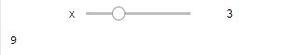Output

The interact() method is used to return the value of the callable in coupling with the sliders. It is real time in nature. The slide in slider will change the value.

### FloatSlider

Like IntSlider, there is a class for float slider which is used to handle the float changes in real time. It is same as IntSlider but it has feature to take steps in float values.

Example:

## Python3

 `import` `ipywidgets as widgets``widgets.interact(``lambda` `x:x``*``*``2``, x ``=` `widgets.FloatSlider(``min` `=` `0``, step ``=``.``25``, ``max` `=` `10``, value ``=` `1``))`

Output: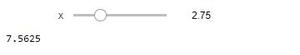Output

### FloatLogSlider

The FloatLogSlider has a log scale, which makes it easy to have a slider that covers a wide range of positive magnitudes. It is generally show to demonstrate the cost values in the machine learning algorithms. In this the min and max refer to the minimum and maximum exponents of the base, and the value refers to the actual value of the slider.

The base parameter allows to alter the log base in the slider.

Example:

## Python3

 `import` `ipywidgets as widgets``widgets.interact(``lambda` `x:x, x ``=` `widgets.FloatLogSlider(description ``=``"\$e ^ x\$"``, ``min` `=` `0``, step ``=` `1``, base ``=` `5``, ``max` `=` `10``, value ``=` `1``))`

Output: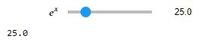Output

### IntRangeSlider

It is the widget used to set a range as an interactive component. It sets a tuple with 2 values the start and the stop values. The Syntax difference with respect to IntSlide is only for the value option.

Example:

## Python3

 `import` `ipywidgets as widgets``widgets.interact(``lambda` `x :x, x ``=` `widgets.IntRangeSlider(``min` `=` `0``, step ``=` `1``, ``max` `=` `10``, value ``=``[``1``, ``2``]))`

Output: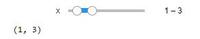Output

### FloatRangeSlider

It is the widget used to set a range as an interactive component. It sets a tuple with 2 values the start and the stop values. The Syntax difference with respect to FloatSlide is only for the value option.

Example:

## Python3

 `import` `ipywidgets as widgets``widgets.interact(``lambda` `x :x, x ``=` `widgets.FloatRangeSlider(``min` `=` `0``, step ``=``.``25``, ``max` `=` `10``, value ``=``[``1``, ``2``]))`

Output: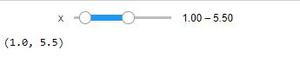Output

My Personal Notes arrow_drop_up